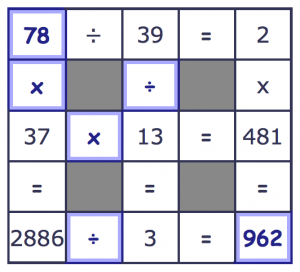# Number Grid PuzzlesMultiplication drills aren't everything, and sometimes a good puzzle is a great way to reinforce those math facts we enjoy practicing so much. These math puzzle worksheets are a great way to work out your basic operator skills, and provide a way to develop number sense as well. There are basic worksheets that cover the fundamental arithmetic operations... Addition and Subtraction PuzzlesMultiplication and Division Puzzles ...as well as more advanced worksheets that mix all four operators... All Operations Puzzles Each series of worksheets includes variations with missing operations, missing numbers, and both. They also increase in difficulty from simple math facts all the way through four digit numbers, and there are variants with negative numbers. The puzzle sample shown here is an answer key for one of the harder multiplication and division puzzle problems. Did you find these puzzles useful? Have any ideas for other puzzle problem worksheets? Drop a note in the comments section below and maybe your worksheet ideas will wind up here in the future... Thanks as always and happy problem solving!